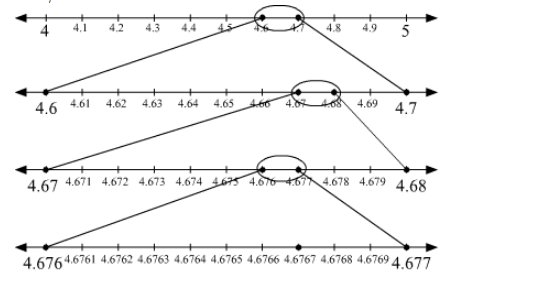Deepak Scored 45->99%ile with Bounce Back Crack Course. You can do it too!

# Visualize the representation of

Question:

Visualize the representation of $4 . \overline{67}$ on the number line up to 4 decimal places.

Solution:

$4 . \overline{67}=4.6767$ (Upto 4 decimal places)

4 < 4.6767 < 5
Divide the gap between 4 and 5 on the number line into 10 equal parts.
Now, 4.6 < 4.6767 < 4.7
In order to locate the point 4.6767 on the number line, divide the gap between 4.6 and 4.7 into 10 equal parts.
Further, 4.67 < 4.6767 < 4.68
To locate the point 4.6767 on the number line, again divide the gap between 4.67 and 4.68 into 10 equal parts.
Again, 4.676 < 4.6767 < 4.677
To locate the point 4.6767 on the number line, again divide the gap between 4.676 and 4.677 into 10 equal parts.
Now, the number 4.6767 can be located on the number line. This can be shown as follows:Here, the marked point represents the point $4 . \overline{67}$ on the number line up to 4 decimal places.